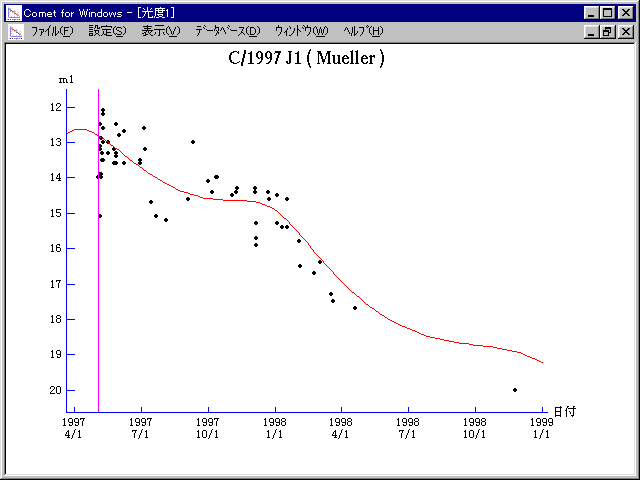# \$B%_%e%i!

C/1997 J1 ( Mueller )###\$B%W%m%U%#!<%k(B

 \$BH/8+F|(B 1997\$BG/(B5\$B7n(B3\$BF|(B \$BH/8+8wEY(B 14\$BEy(B \$BH/8+ Jean Mueller

###\$B50F;MWAG(B

```   The following improved orbital elements by Kenji Muraoka, are
from 479 observations 1997 May 3 to 1998 Nov. 25, perturbations
by 9 Planets, Moon and 5 minor planets were taken into account.
The mean residual is +/- 0.57 arc seconds.

Epoch  =  1997 Apr. 22.0  TT       JDT = 2450560.5
T  =  1997 May   3.80219       +/- 0.00019 (m.e.) TT
Peri. =   98.95424                +/- 0.00011
Node  =  277.07493                +/- 0.00007   (2000.0)
Incl. =  122.96869                +/- 0.00003
q  =    2.3021499              +/- 0.0000027 AU
e  =    0.9908918              +/- 0.0000026
1/a  =   +0.0039564              +/- 0.0000011 1/AU
( P  =   4018 years )
orig. 1/a  =   +0.001823   1/AU
fut.  1/a  =   +0.004661   1/AU
```

###\$B@1?^(B1996\$BG/(B10\$B7n(B 4\$BF|!A(B1997\$BG/(B11\$B7n(B28\$BF|(B1997\$BG/(B 6\$B7n(B21\$BF|!A(B1998\$BG/(B12\$B7n(B13\$BF|(B

###\$B8wEYJQ2=(B

```        m1 = 7.5 + 5 log\$B&\$(B + 10.0 log r
```##### \$B50F;MWAG\$OB<2,7r<#;a\$N7W;;\$K\$h\$k\$b\$N\$G\$9!#(B \$B@1?^\$O(B StellaNavigator Ver.2.0 for Windows (\$B%"%9%H%m%"!<%D(B \$BJTCx(B / \$B%"%9%-!<=PHG6I4)(B) \$B\$G:n@.\$7\$?\$b\$N\$G\$9!#(B \$B8wEY%0%i%U\$O(BComet for Windows\$B\$G:n@.\$7\$?\$b\$N\$G\$9!#(B